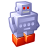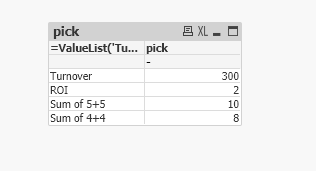# QlikView App Dev

Discussion Board for collaboration related to QlikView App Development.

Announcements
Join this live chat April 6, 10AM EST - QlikView to Qlik Sense REGISTER
cancel
Showing results for
Search instead for
Did you mean:Contributor III

## Generate formulas in table

Hello,
I try to create an overview of key figures in a table. This could be a pivot table, for example. I still need two comment fields for the key figure. This table can look like this:

 KPI Comment1 Comment 2 Formula turnover a a 2.015.200 ROI s s 215.000 Sum of 5+5 d d 10 Sum of 4+4 f f 8

The Formula column is to be calculated from different QlikView fields with different formulas. Does anyone know a good solution for this?

My idea is to load a new table for key figures into the data model. I create the new table in an Excel spreadsheet. In this table I define all columns and write the formulas I need. This will look like this.

 KPI Comment1 Comment 2 Formula turnover a a =sum(turnover) ROI s s =sum(NetIncome)/sum(Investment) Sum of 5+5 d d =5+5 Sum of 4+4 f f =4+4

Now I have created the table in QlikView. For this I created a pivot with the columns KPI, Comment1 and Comment2. As formula I use the following function:

\$(=Formula)

If I filter the table to one KPI it works. With several formulas this doesn't work anymore. Does anyone have an idea what I did wrong?

I hope someone of you can help me.
Greetings
Alex

Labels (3)

• ### QlikView

1 Solution

Accepted SolutionsPartner

Hi,

You'd have to get a calculated dimension with ValueList like so:

=ValueList('Turnover','ROI','Sum of 5+5','Sum of 4+4')

This will create a dummy dimension with 4 text on it.
As for expression, you'd use:

pick
(
match
(
ValueList('Turnover','ROI','Sum of 5+5','Sum of 4+4'),
'Turnover',
'ROI',
'Sum of 5+5',
'Sum of 4+4'
),
\$(v1),
\$(v2),
\$(v3),
\$(v4)
)

Ive created the v1,v2,v3 and v4 variables like so:
v1: sum(value)
v2: 2
v3: 5+5
v4: 4+4

Resulting in:1 ReplyPartner

Hi,

You'd have to get a calculated dimension with ValueList like so:

=ValueList('Turnover','ROI','Sum of 5+5','Sum of 4+4')

This will create a dummy dimension with 4 text on it.
As for expression, you'd use:

pick
(
match
(
ValueList('Turnover','ROI','Sum of 5+5','Sum of 4+4'),
'Turnover',
'ROI',
'Sum of 5+5',
'Sum of 4+4'
),
\$(v1),
\$(v2),
\$(v3),
\$(v4)
)

Ive created the v1,v2,v3 and v4 variables like so:
v1: sum(value)
v2: 2
v3: 5+5
v4: 4+4

Resulting in: